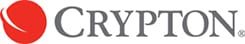# Crypton RevlA whole new spin on coated fabrics.

• Superior clean-ability
• Unmatched stain resistance
• Extreme durability
• Antimicrobial
• Beauty and longevity
• Wide array of colours
• Attached is PDF  that shows a list of cleaning products and disinfectants, which have been tested and approved by Crypton for Crypton Revl

Cleaners & Disinfectants Acceptable List – Crypton Revl

Array ( [name] => [pa_collection] => [usage] => [pa_repeat] => [pattern-repeat] => [pattern-repeat-textiles] => [pattern-repeat-wall-covering] => [wv-pattern-repeat] => [textile-repeat] => [pa-pattern-repeat] => [printed-width] => [pa_printed-width] => [pa_width] => [pa_roll-length] => [roll-length] => [pa_flammability] => [installationcare] => [pa_application] => [special-notes] => [pa_style] => [pa_composition] => [pa_colourway] => ) Array ( [pa_colourway] => WC_Product_Attribute Object ( [data:protected] => Array ( [id] => 6 [name] => pa_colourway [options] => Array (  => 418  => 903  => 319  => 1591  => 1590  => 1493  => 223  => 654  => 738  => 294  => 1592  => 225  => 538  => 919  => 297  => 226  => 276  => 453  => 228  => 1593  => 314  => 1279  => 1589 ) [position] => 0 [visible] => [variation] => 1 ) ) [pa_colour] => WC_Product_Attribute Object ( [data:protected] => Array ( [id] => 9 [name] => pa_colour [options] => Array (  => 138  => 140  => 137  => 163  => 141  => 162  => 769  => 211  => 139  => 142  => 143  => 378  => 210  => 179  => 144  => 145  => 146  => 147  => 148  => 150  => 149 ) [position] => 1 [visible] => [variation] => ) ) [pa_width] => WC_Product_Attribute Object ( [data:protected] => Array ( [id] => 1 [name] => pa_width [options] => Array (  => 172 ) [position] => 2 [visible] => 1 [variation] => ) ) [pa_textile-repeat] => WC_Product_Attribute Object ( [data:protected] => Array ( [id] => 2 [name] => pa_textile-repeat [options] => Array (  => 203 ) [position] => 3 [visible] => 1 [variation] => ) ) [pa_flammability] => WC_Product_Attribute Object ( [data:protected] => Array ( [id] => 4 [name] => pa_flammability [options] => Array (  => 1597 ) [position] => 4 [visible] => 1 [variation] => ) ) [pa_usage] => WC_Product_Attribute Object ( [data:protected] => Array ( [id] => 5 [name] => pa_usage [options] => Array (  => 1398  => 1401 ) [position] => 5 [visible] => 1 [variation] => ) ) [pa_application] => WC_Product_Attribute Object ( [data:protected] => Array ( [id] => 10 [name] => pa_application [options] => Array (  => 1454  => 1453  => 1438  => 1441 ) [position] => 6 [visible] => [variation] => ) ) [pa_style] => WC_Product_Attribute Object ( [data:protected] => Array ( [id] => 8 [name] => pa_style [options] => Array (  => 176 ) [position] => 7 [visible] => [variation] => ) ) [pa_composition] => WC_Product_Attribute Object ( [data:protected] => Array ( [id] => 3 [name] => pa_composition [options] => Array (  => 1595 ) [position] => 8 [visible] => 1 [variation] => ) ) [pa_collection] => WC_Product_Attribute Object ( [data:protected] => Array ( [id] => 7 [name] => pa_collection [options] => Array (  => 70 ) [position] => 9 [visible] => 1 [variation] => ) ) ) Array ( [name] => [pa_collection] => WC_Product_Attribute Object ( [data:protected] => Array ( [id] => 7 [name] => pa_collection [options] => Array (  => 70 ) [position] => 9 [visible] => 1 [variation] => ) ) [usage] => [pa_repeat] => [pattern-repeat] => [pattern-repeat-textiles] => [pattern-repeat-wall-covering] => [wv-pattern-repeat] => [textile-repeat] => [pa-pattern-repeat] => [printed-width] => [pa_printed-width] => [pa_width] => WC_Product_Attribute Object ( [data:protected] => Array ( [id] => 1 [name] => pa_width [options] => Array (  => 172 ) [position] => 2 [visible] => 1 [variation] => ) ) [pa_roll-length] => [roll-length] => [pa_flammability] => WC_Product_Attribute Object ( [data:protected] => Array ( [id] => 4 [name] => pa_flammability [options] => Array (  => 1597 ) [position] => 4 [visible] => 1 [variation] => ) ) [installationcare] => [pa_application] => WC_Product_Attribute Object ( [data:protected] => Array ( [id] => 10 [name] => pa_application [options] => Array (  => 1454  => 1453  => 1438  => 1441 ) [position] => 6 [visible] => [variation] => ) ) [special-notes] => [pa_style] => WC_Product_Attribute Object ( [data:protected] => Array ( [id] => 8 [name] => pa_style [options] => Array (  => 176 ) [position] => 7 [visible] => [variation] => ) ) [pa_composition] => WC_Product_Attribute Object ( [data:protected] => Array ( [id] => 3 [name] => pa_composition [options] => Array (  => 1595 ) [position] => 8 [visible] => 1 [variation] => ) ) [pa_colourway] => WC_Product_Attribute Object ( [data:protected] => Array ( [id] => 6 [name] => pa_colourway [options] => Array (  => 418  => 903  => 319  => 1591  => 1590  => 1493  => 223  => 654  => 738  => 294  => 1592  => 225  => 538  => 919  => 297  => 226  => 276  => 453  => 228  => 1593  => 314  => 1279  => 1589 ) [position] => 0 [visible] => [variation] => 1 ) ) [pa_colour] => WC_Product_Attribute Object ( [data:protected] => Array ( [id] => 9 [name] => pa_colour [options] => Array (  => 138  => 140  => 137  => 163  => 141  => 162  => 769  => 211  => 139  => 142  => 143  => 378  => 210  => 179  => 144  => 145  => 146  => 147  => 148  => 150  => 149 ) [position] => 1 [visible] => [variation] => ) ) [pa_textile-repeat] => WC_Product_Attribute Object ( [data:protected] => Array ( [id] => 2 [name] => pa_textile-repeat [options] => Array (  => 203 ) [position] => 3 [visible] => 1 [variation] => ) ) [pa_usage] => WC_Product_Attribute Object ( [data:protected] => Array ( [id] => 5 [name] => pa_usage [options] => Array (  => 1398  => 1401 ) [position] => 5 [visible] => 1 [variation] => ) ) )

Collection: Crypton

Width: 137cm approx.

Flammability: AS/NZS 1530 pt 3, CAL 117E – 2013, NFPA 260, IMO 210 FTP Code Section 3.1 & 3.2

Composition: Face :100% PVC, Backing:100% Polyester/Cotton knit

Usage: Waterproof Upholstery, Upholstery